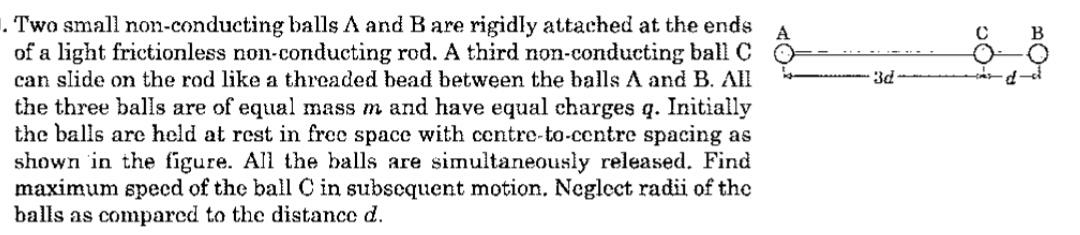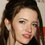# 3 Charges and unsuccessful attempt

Here is a problem , which I was solving todayHere is my attempt:
I think the toral electrostatic energy of system will be conversed.
It will interchange between kinetic energy and electrostatic potential energy.
This is starting energy of system$E_{Total }=\frac{19}{12} \frac{Kq^{2}}{d}$
After that the net external force in the system is zero.
Si the centre of mass will be in constant, and will lie in x axis only [x_{com}=\frac{4d}{3}]
When the system is released , force will start acting simultaneously on all 3 charge, therefore all will acquire different velolicty in different direction.
Let the velocity of 1st, 2nd, 3rd charge be $\vec{v_{1}},\vec{ v_{2}}, \vec{v_{3}}$ .
No forces is acting on the system , so at any time the velocity of center of mass will be zero$v_{com}=\frac{m \vec{v_{1}}+m \vec{v_{2}}+m \vec{v_{3}}}{m+m+m}=0$
$\vec{v_{1}}+\vec{v_{2}}+ \vec{v_{3}}=0$ Now, how to proceed?

Share your views.
Thanks in advance.Note by Talulah Riley
9 months ago

This discussion board is a place to discuss our Daily Challenges and the math and science related to those challenges. Explanations are more than just a solution — they should explain the steps and thinking strategies that you used to obtain the solution. Comments should further the discussion of math and science.

When posting on Brilliant:

• Use the emojis to react to an explanation, whether you're congratulating a job well done , or just really confused .
• Ask specific questions about the challenge or the steps in somebody's explanation. Well-posed questions can add a lot to the discussion, but posting "I don't understand!" doesn't help anyone.
• Try to contribute something new to the discussion, whether it is an extension, generalization or other idea related to the challenge.
• Stay on topic — we're all here to learn more about math and science, not to hear about your favorite get-rich-quick scheme or current world events.

MarkdownAppears as
*italics* or _italics_ italics
**bold** or __bold__ bold
- bulleted- list
• bulleted
• list
1. numbered2. list
1. numbered
2. list
Note: you must add a full line of space before and after lists for them to show up correctly
paragraph 1paragraph 2

paragraph 1

paragraph 2

[example link](https://brilliant.org)example link
> This is a quote
This is a quote
    # I indented these lines
# 4 spaces, and now they show
# up as a code block.

print "hello world"
# I indented these lines
# 4 spaces, and now they show
# up as a code block.

print "hello world"
MathAppears as
Remember to wrap math in $$ ... $$ or $ ... $ to ensure proper formatting.
2 \times 3 $2 \times 3$
2^{34} $2^{34}$
a_{i-1} $a_{i-1}$
\frac{2}{3} $\frac{2}{3}$
\sqrt{2} $\sqrt{2}$
\sum_{i=1}^3 $\sum_{i=1}^3$
\sin \theta $\sin \theta$
\boxed{123} $\boxed{123}$

## Comments

Sort by:

Top Newest

@Steven Chase @Karan Chatrath @Uros Stojkovic Your inputs would be helpful.

- 9 months ago

Log in to reply

I'll try the problem soon, despite the fact that you already have a solution.

- 9 months ago

Log in to reply

@Krishna Karthik in case you are interested.

- 9 months ago

Log in to reply

Is the answer :

$\sqrt{\frac{q^2}{9 \pi \epsilon_o md}}$

- 9 months ago

Log in to reply

@Karan Chatrath Yes you are $\Huge CORRECT$

- 9 months ago

Log in to reply

@Karan Chatrath please share your solution

- 9 months ago

Log in to reply

The above note is edited.

- 9 months ago

Log in to reply

Let the X coordinate of the leftmost charge be $x_1=x$, that of the middle charge be $x_2=x+s$ and that of the rightmost charge be $x_3=x +4d$. Applying Newton's second law to each of the masses gives the following:

$m\ddot{x}_1 = -\frac{kq^2}{s^2} -\frac{kq^2}{16d^2}$ $m\ddot{x}_2 = \frac{kq^2}{s^2} -\frac{kq^2}{(4d-s)^2}$ $m\ddot{x}_3 = \frac{kq^2}{16d^2} +\frac{kq^2}{(4d-s)^2}$

Adding all of the above equations gives:

$\ddot{x}_1 + \ddot{x}_2 + \ddot{x}_3 =0$ $\implies 3\ddot{x} + \ddot{s} = 0$

$\implies \dot{x} = -\frac{\dot{s}}{3}$

Plugging this result in the 2nd equation of motion gives:

$\ddot{s} = \frac{3kq^2}{2}\left(\frac{1}{s^2} - \frac{1}{(4d-s)^2} \right)$

$s(0) = 3d$ $\dot{s}(0) = 0$

The speed of the second particle is:

$\dot{x}_2 = \dot{x}+\dot{s} = \frac{2\dot{s}}{3}$

Can you proceed from here?

- 9 months ago

Log in to reply

Edited comment above to remove typos. Hope this helps.

- 9 months ago

Log in to reply

@Karan Chatrath thanks, let me try to proceed.

- 9 months ago

Log in to reply

@Karan Chatrath I just found an alternative approch, it was a very question also.
Basically I thought that author wants to say that at $t=0$ all balls are released freely.
But after $t=0$ , extreme balls are still rigidly attached.
Here is an alternative method:
Electrostatic energy $E=\frac{Kq^{2}}{s}+\frac{Kq^{2}}{(4d-s)}+\frac{Kq^{2}}{4d}$

Whenever that middle ball will acquire maximum velocity, at that instant will have minimum energy.
$\frac{dE}{ds}=0$
After solving above equation gives $\boxed{s=2d}$

I am posting a new note within a hour showing a another attempt of a problem.
Thanks in advance.

- 9 months ago

Log in to reply

Nice alternative method. I will look at your other note later.

- 9 months ago

Log in to reply

×

Problem Loading...

Note Loading...

Set Loading...